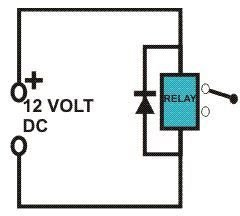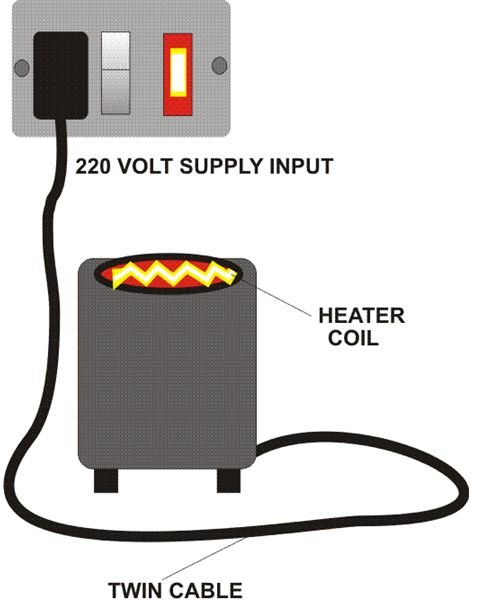# What is Circuit Analysis? Basic Theory Expounded

## Introduction

It would be absolutely absurd and a dangerous idea for anybody thinking of going for a deep sea exploration without first learning how to swim. The same is true in the field of electrical engineering and electronics. Without a thorough knowledge of the basics, success in the relevant fields may be quite farfetched.

In this article we will go through a detailed study of a few circuit configurations and try to solve the problems involved in these elementary electrical circuits through illustrative examples.

Let’s proceed and find out exactly what circuit analysis is.

## What is Circuit Analysis?

In an electrical circuit the process of studying and analyzing the various electrical quantities involved, especially the nodal voltages and currents through calculations, is known as circuit analysis.

To be able to solve the various problems generally involved in practical electrical circuits, it would be first important to learn the following basic units of electricity:

Current (I): The unit of current is Ampere (A) and is defined as the quantity of current forced by a pressure of one volt through a resistance of one Ohm.

Voltage (V): The unit of voltage is Volt (V) and is defined as amount of pressure required to force a current of one ampere through a resistance of one Ohm.

While talking of voltages we should be careful about the following two terms:

• Electromotive Force (E): It is the voltage that exists across the terminals of a battery or dynamo which is not connected to any external circuit.
• Potential Difference (U): It is the voltage that exists across the terminals of a battery or dynamo that is connected to an external circuit. It may be calculated using the below given formula:

U = (E – Internal resistance of battery or dynamo) × I

Resistance (): The unit of resistance is Ohm (Ω) and is defined as the amount of resistance offered by a circuit so that a current of one ampere is allowed to flow through it at a pressure of one volt.

## Ohm’s Law

According to Ohm’s Law Current (I) = Voltage (U) ÷ Resistance (Ω)

Thus through Ohm’s law we are able to get a clear relationship between current, voltage and resistance.

Let’s try to understand it by solving the below given simple problem.Q: Referring to the figure, calculate the amount of current flowing through the relay coil having a resistance of 400 Ohms and a potential difference of 12 volts across it.

A: Using Ohm’s law I = U/R = 12/ 400 = 0.03 Ampere or 0.03 × 1000 = 30 milliAmpere(mA)

## How to Solve Circuit Calculations Consisting of Series and Parallel Resistances

Resistances in series may be simply calculated by adding their values together and resistances in parallel may be calculated by adding the reciprocals of each resistor and then dividing 1 by the result.

Let’s solve this simple circuit problem very commonly asked in different basic engineering circuit analysis questions:Q: Referring to the adjoining figure, calculate the equivalent resistance and the current flowing through the circuit having a potential difference of 12 volts.

A: First the total value of the resistances connected in parallel is calculated as: 1/R1+1/R2+1/R3 = 1/0.5+1/0.5+1/0.5 = 6, dividing 1 by 6 gives 0.16 Ohms.

Now since the above calculated value is in series with the resistor R4, the final value can be simply calculated as: 0.16 + 5 = 5.16 Ohms.

Therefore using Ohm’s law the current passing through the circuit is:

I = U/R = 12/5.16 = 2.32 Amperes.

Similarly in any given circuit the potential difference or the resistance can also be calculated by using Ohm’s law.

Let’s move ahead and study few other parameters generally involved in slightly more complicated electrical circuits.

## How to Calculate the Resistance of Metallic Cables?

In basic engineering circuit analysis it sometimes becomes very important to know the resistance of a conductor, for example the mains cable. Let’s see how it’s calculated:

Resistance of Metallic Conductors: It may be calculated using the following simple formula:

R = ρ×l/a

where R = Resistance in Ohms, ρ = Resistivity of the conductor material, l = Length of the conductor and a = cross sectional area of the conductor.

The resistivity of the material is actually the resistance present in a meter long conductor having a cross sectional area of 1mm2 and is constant.

Electrical Power: Its unit is Watt and is defined as the product of current and voltage.

Hence W = IU = I2R, (by replacing U = IR).

The following given problem and its solution will help us to understand the use of the above discussed two electrical parameters:Q: Referring to the adjoining figure, an electrical heater consuming 6 amperes of current is connected to a 220 volts supply by a copper cable having a length of 12 meters and a diameter of 1.5 mm2. Calculate the resistance of the cable and also the power consumption of the appliance. (ρ of copper is 0.017 Ωm).

A: First let’s find out the resistance of the conductor i.e. the cable as:

R = ρ×l/a = 0.017 × 12/1.5 = 0.136, since the cable is made up of two wires, multiplying the result by 2 we get the final result as 0.272 Ohms.

The Power of the heater can be easily obtained in the following manner:

P = I × U = 6 × 220 = 1320 Watts.

Through the above explanations we have perhaps just been able to scratch the surface and a comprehensive answer to the question “What is basic engineering circuit analysis?” can be so huge that it would fill volumes, however, hopefully you should be able to learn much more regarding this through my future articles.